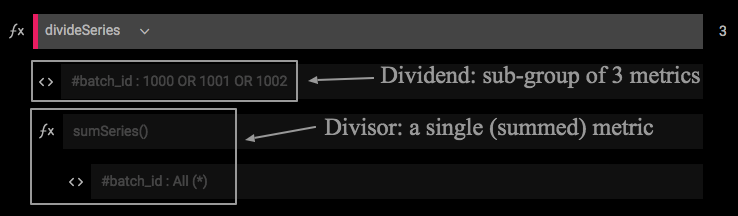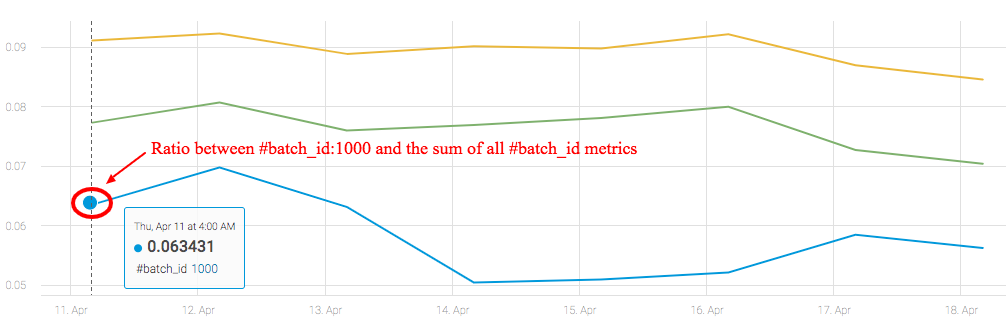DivideSeries

 Description Returns the division result of each sample in the dividend time-series divided by the corresponding sample in the divisor time-series.  See also ratioPairs for getting the ratio between multiple pairs of metrics. Input Dividend Time Series: Multiple (Single or multiple time-series) Divisor Time Series: Single Parameters None Output Transformed Time Series: Multiple Available in Alerts, Composites, Dashboards

The divideSeries function provides the most straightforward way to divide a group of time-series by a single time-series. This is achieved by entering two metric expressions; one as the dividend, and another as the divisor. Importantly, the divisor must hold a single time-series.

Usage Example

ThedivideSeries function can be used when you want to monitor the ratio between one (or more) time-series and some quantity (such as the total sum of a group of time-series):The result of the composite above is a group of three metrics, each one holding the calculated ratios between a specific #batch_id metric and the total sum of all #batch_id metrics:Note: to divide matching pairs of time-series, see ratioPairs.